Function Repository Resource:

# LensManipulate

Generate a graphic in which the thin-lens parameters can be manipulated dynamically

Contributed by: Julián Laverde
Jason Martinez
 ResourceFunction["LensManipulate"][] returns a manipulable graphic of the ray diagram of a thin lens. ResourceFunction["LensManipulate"][assoc] returns a graphic with the corresponding parameters in the Association assoc.

## Details and Options

The available thin lenses are biconvex and biconcave.
ResourceFunction["LensManipulate"] follows the sign convention for thin lenses from Hecht, Optics, Pearson, 2015:
 Object distance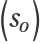(+) real object (-) virtual object Focal length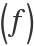(+) converging lens (-) diverging lens Image distance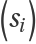(+) real image (-) virtual image Object size (+) erect object (-) inverted object Image size (+) erect image (-) inverted image Magnification (M) (+) erect image (-) inverted image
The linear magnification factor of the system is given by the equation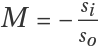, defined as the ratio of the size of the image compared to the size of the object.
ResourceFunction["LensManipulate"] uses the Gaussian lens formula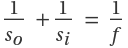to calculate the image distance si.
The arrow in the middle of the rays represents the direction of light and dotted lines the projection of the rays.
Real images and virtual images are represented by a thick-line arrow and a dotted-line arrow, respectively.
In ResourceFunction["LensManipulate"][assoc], the Association assoc can contain the following elements:
 "Diameter" 4 lens (half) diameter "ObjectSize" 0.5 object initial size "FocalLength" "Diameter" lens initial focal length "ObjectDistance" "FocalLength" object initial position
"ObjectDistance" can be a positive number or quantity of length.
"ObjectSize" can be a number or quantity of length in the range[-1,1].
"FocalLength" can be a positive or negative number or quantity of length.
Quantity inputs should have units of length. Results and values in sliders will be shown in the most common unit among the inputs.
ResourceFunction["LensManipulate"][] takes the options of Graphics and the following:
 "RayColor" Automatic specify the colors of the rays "LensColor" Automatic specify the color of the lens "ArrowColor" Automatic specify the colors of the object and image arrows "ShowEquations" True set the equations in the graphics object "ObjectDistanceRange" Automatic specify the range of "ObjectDistance" "ObjectSizeRange" Automatic specify the range of "ObjectSize" "FocalLengthRange" Automatic specify the range of "FocalLength"
The copy values button copies the current values of "ObjectDistance", "FocalLength", "ImageDistance" and "Magnification" to the clipboard as an Association.

## Examples

### Basic Examples (2)

Get a manipulable thin lens ray diagram:

 In:=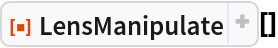Out=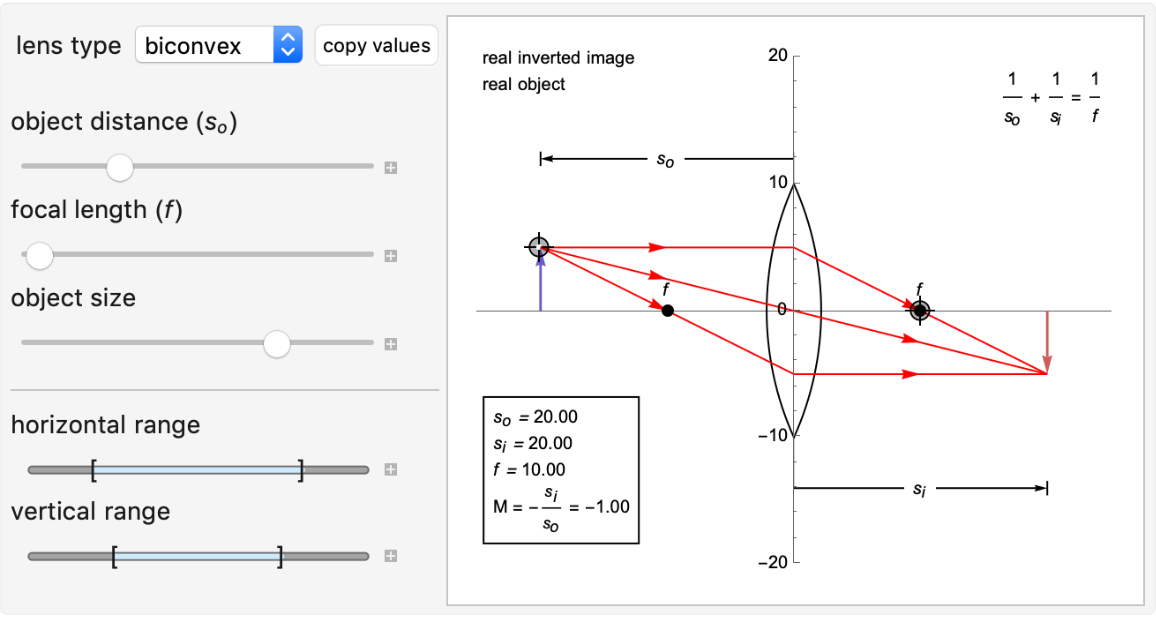Get the ray diagram for a set of specified initial values:

 In:=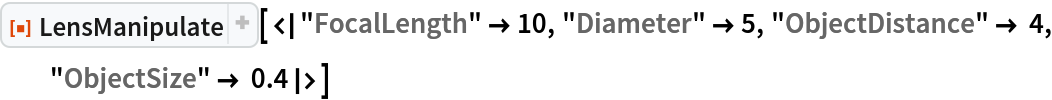Out=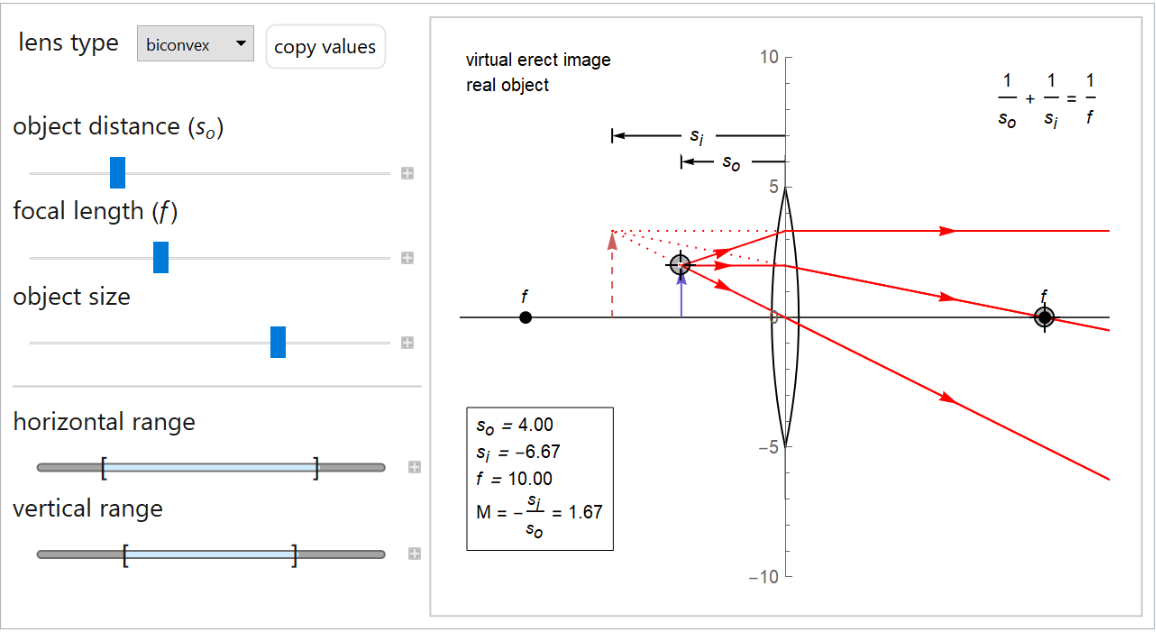### Scope (2)

Get the ray diagram for a negative thin lens:

 In:=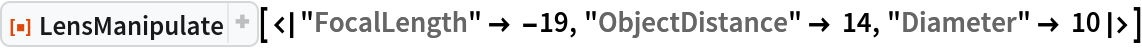Out=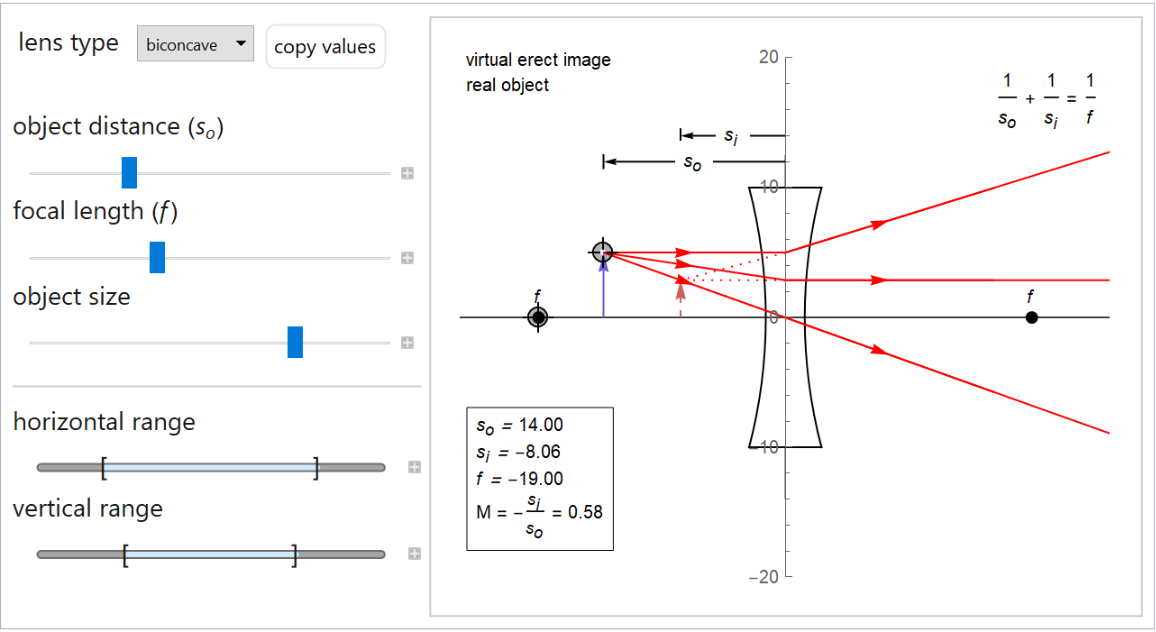Include inputs with quantities:

 In:=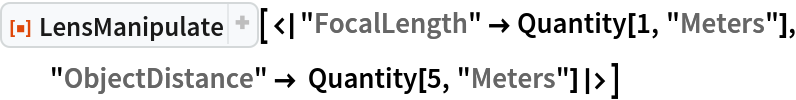Out=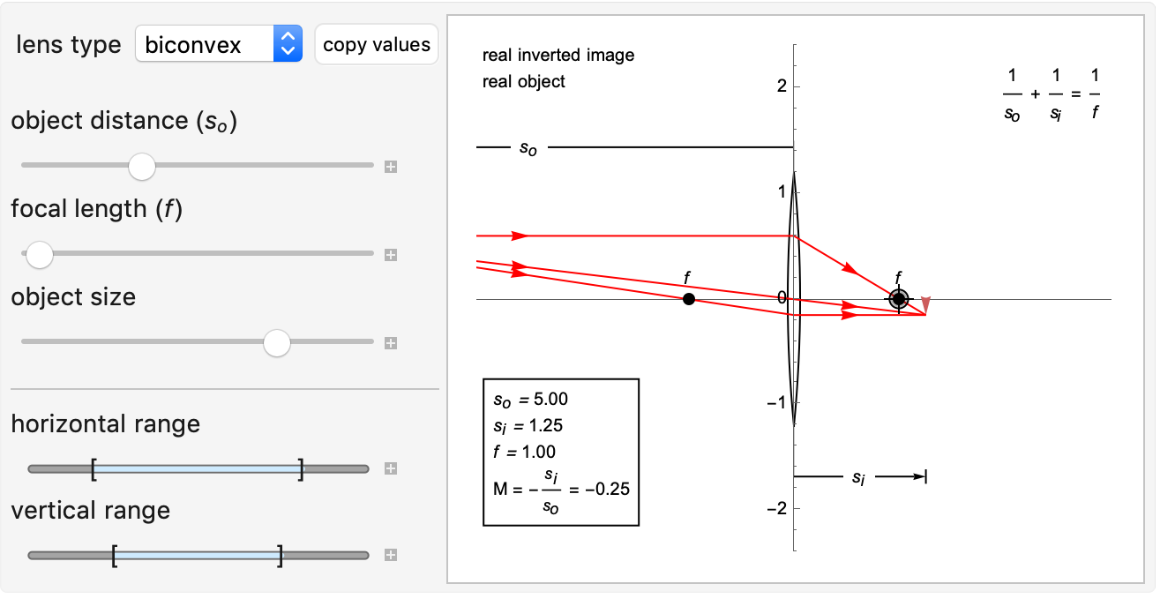### Options (7)

LensManipulate takes the options of Graphics:

 In:=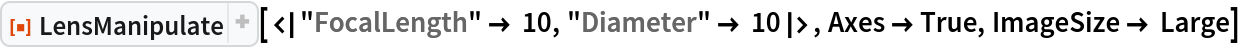Out=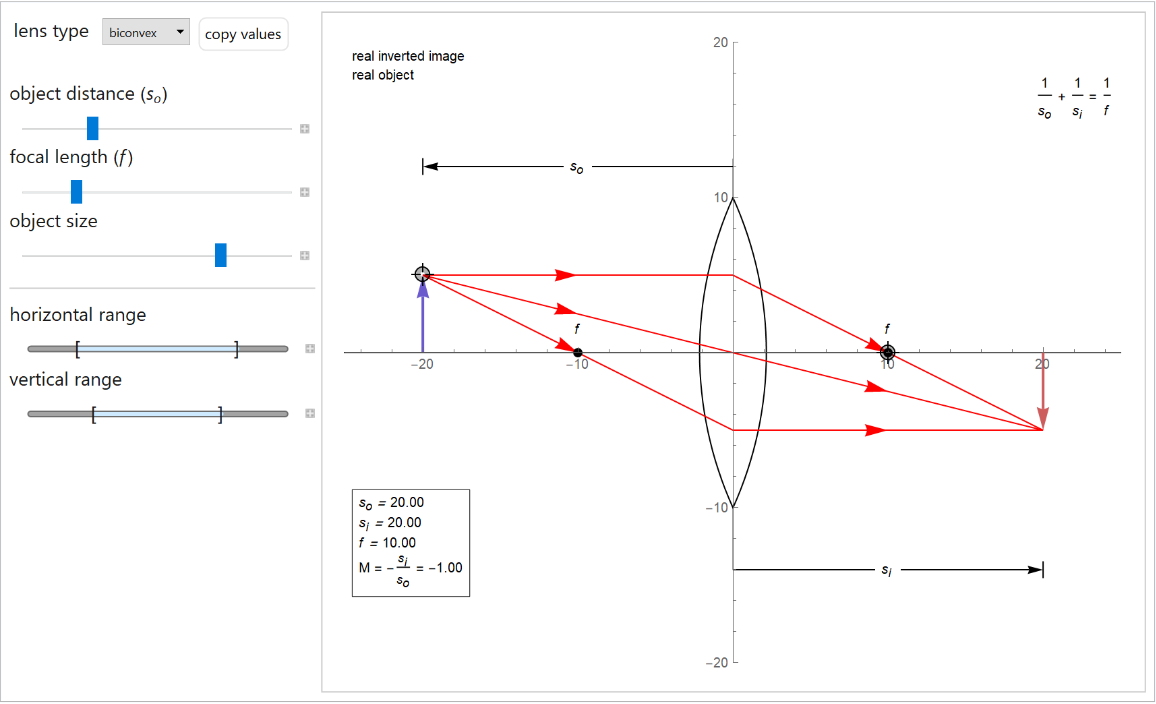#### ShowEquations (1)

Set "ShowEquations" to False:

 In:=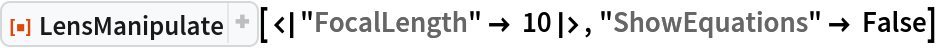Out=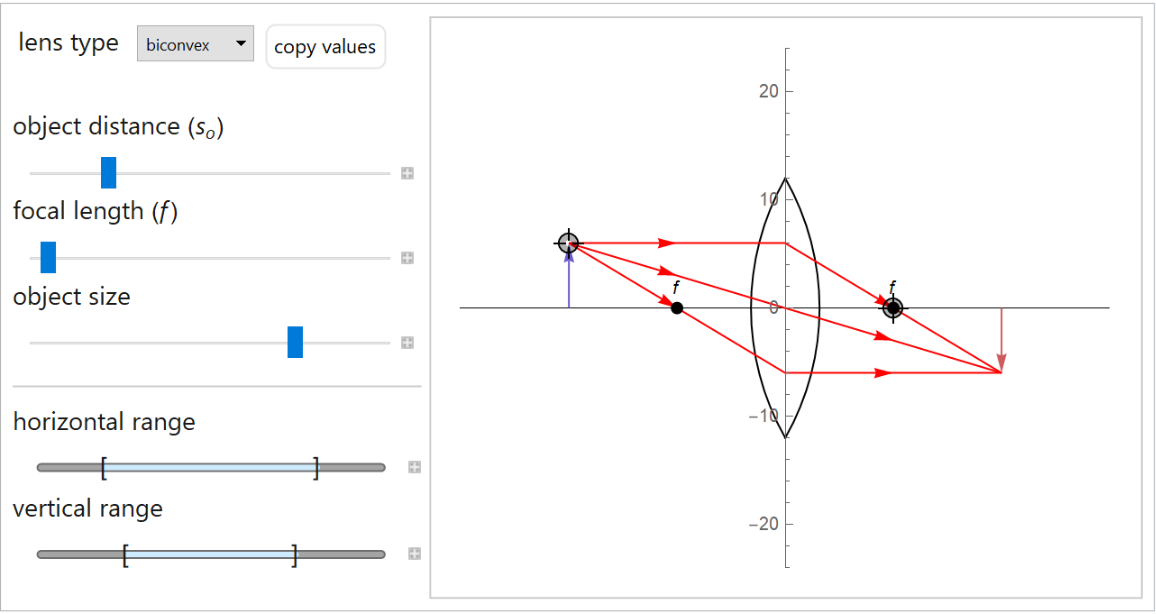#### RayColor (1)

Change the color of the rays:

 In:=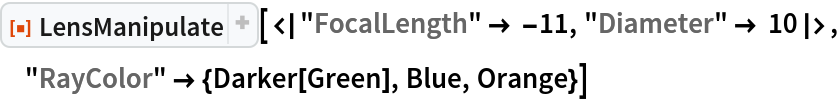Out=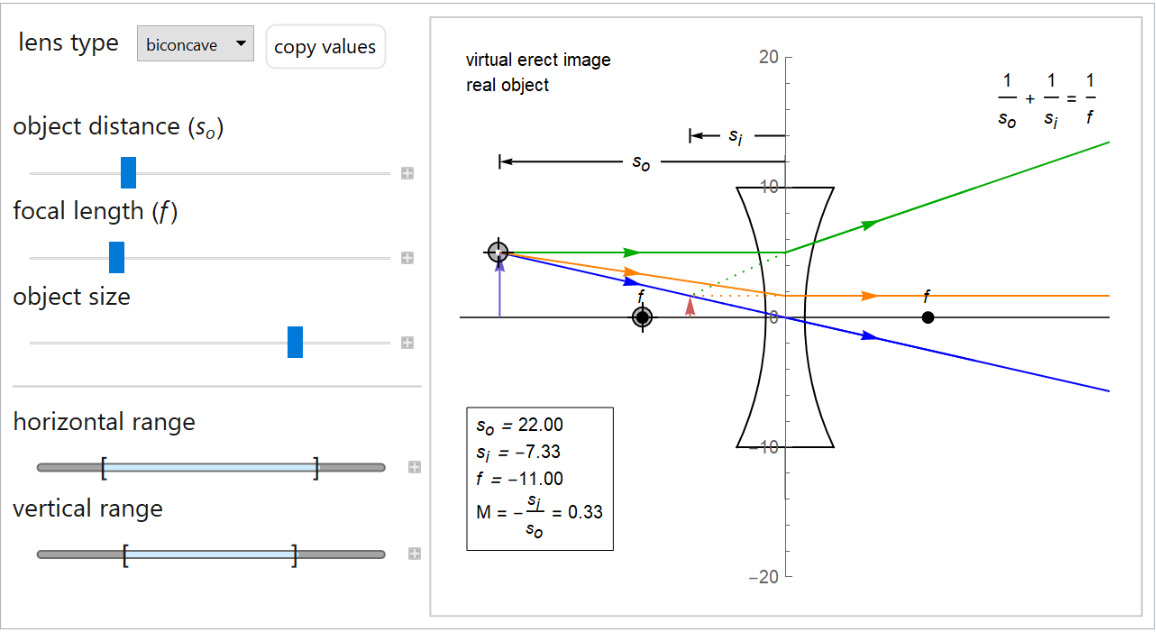#### ArrowColor (1)

Change the color of the object and virtual arrows:

 In:=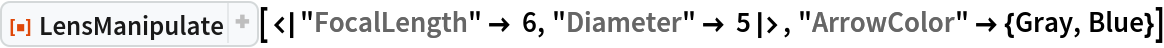Out=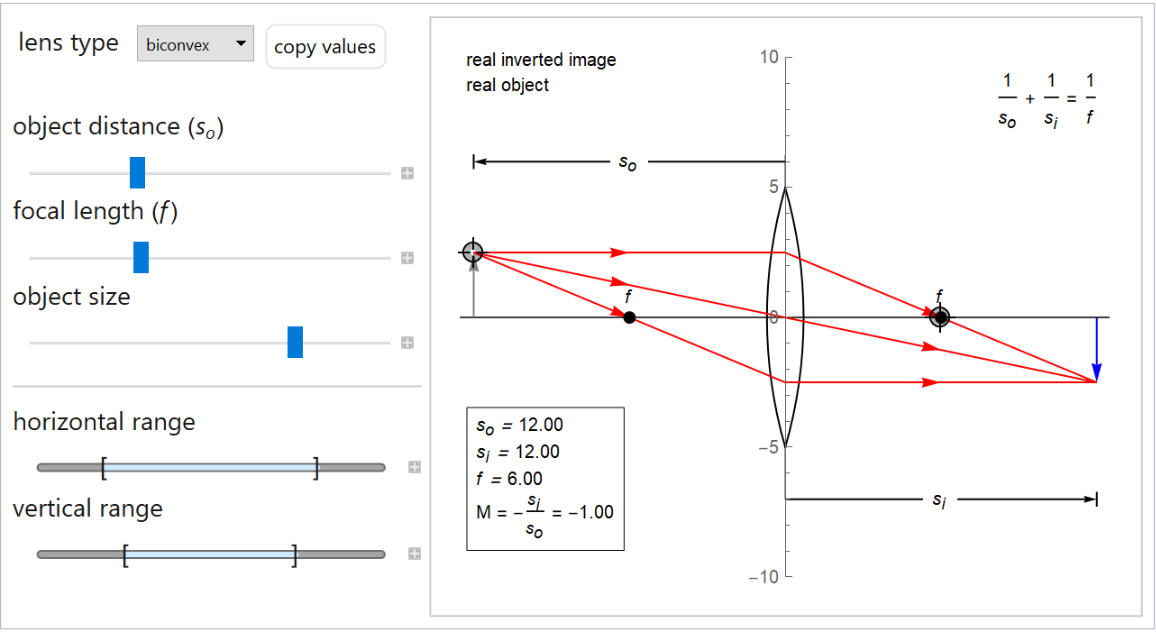#### LensColor (1)

Specify the color of the lens:

 In:=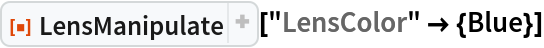Out=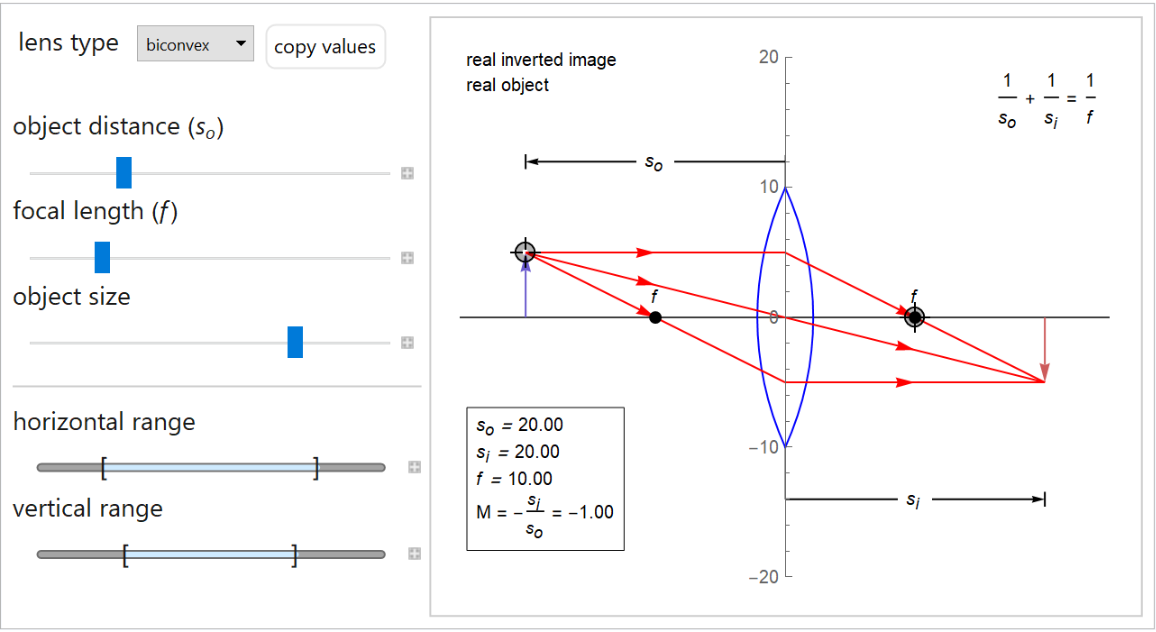#### ObjectDistanceRange (1)

Specify the range of "ObjectDistance":

 In:=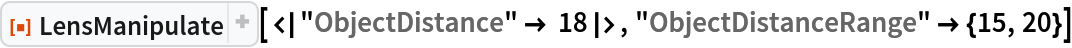Out=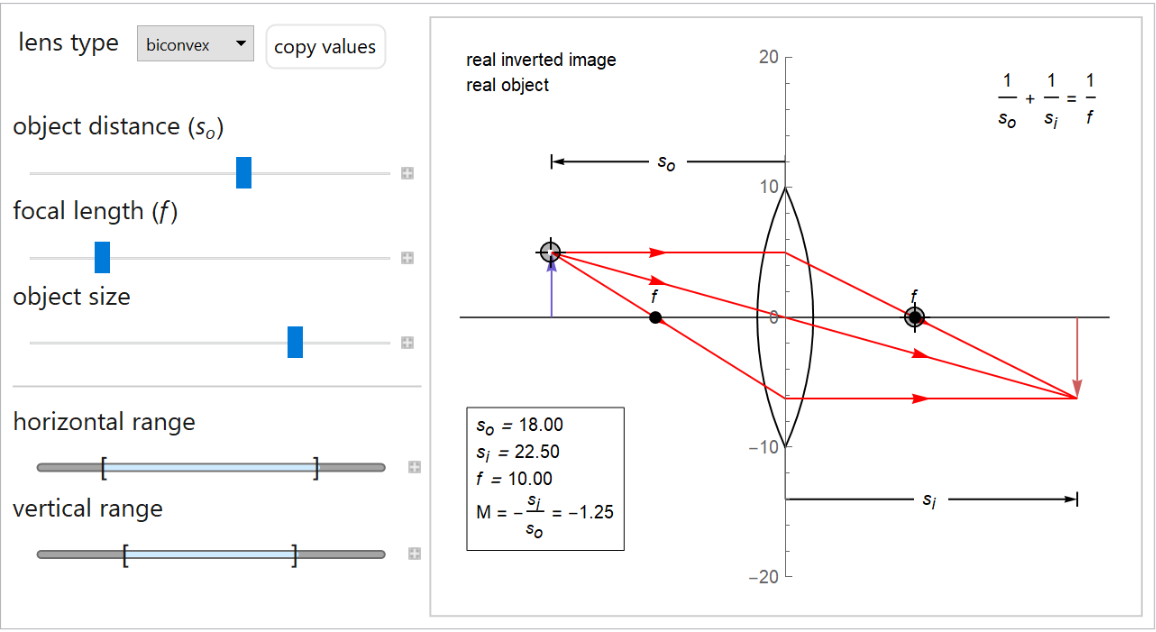#### ObjectSizeRange (1)

Specify the range of "ObjectSize":

 In:=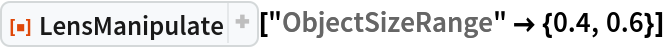Out=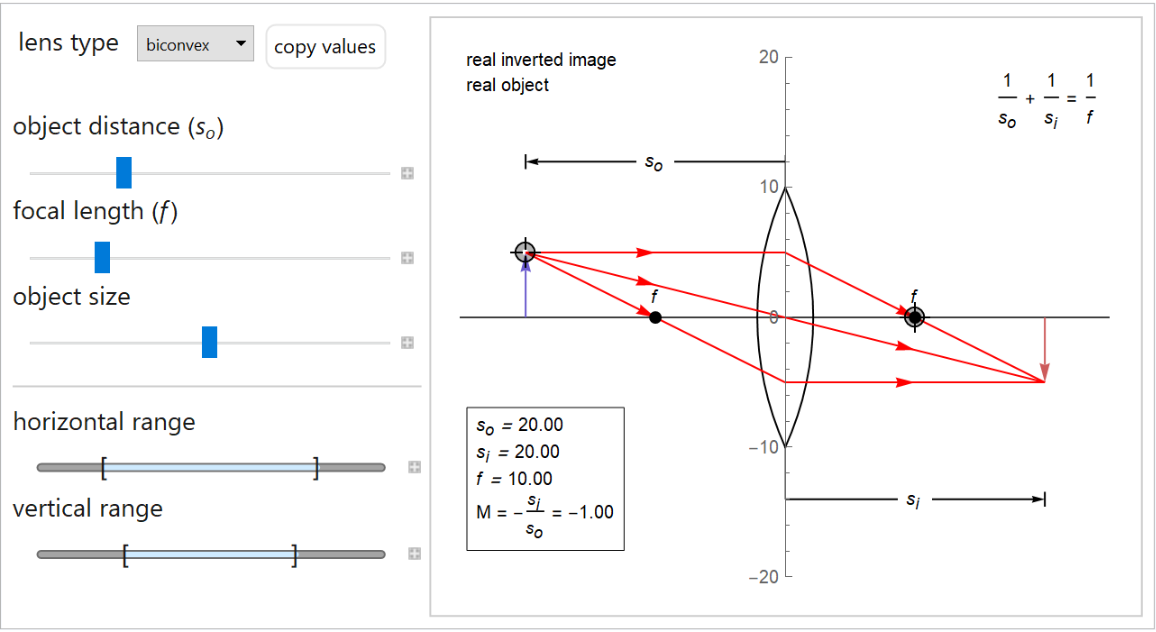#### FocalLengthRange (1)

Specify the range of "FocalLength":

 In:=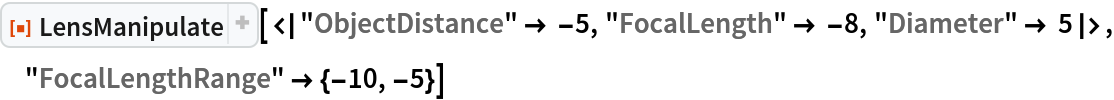Out=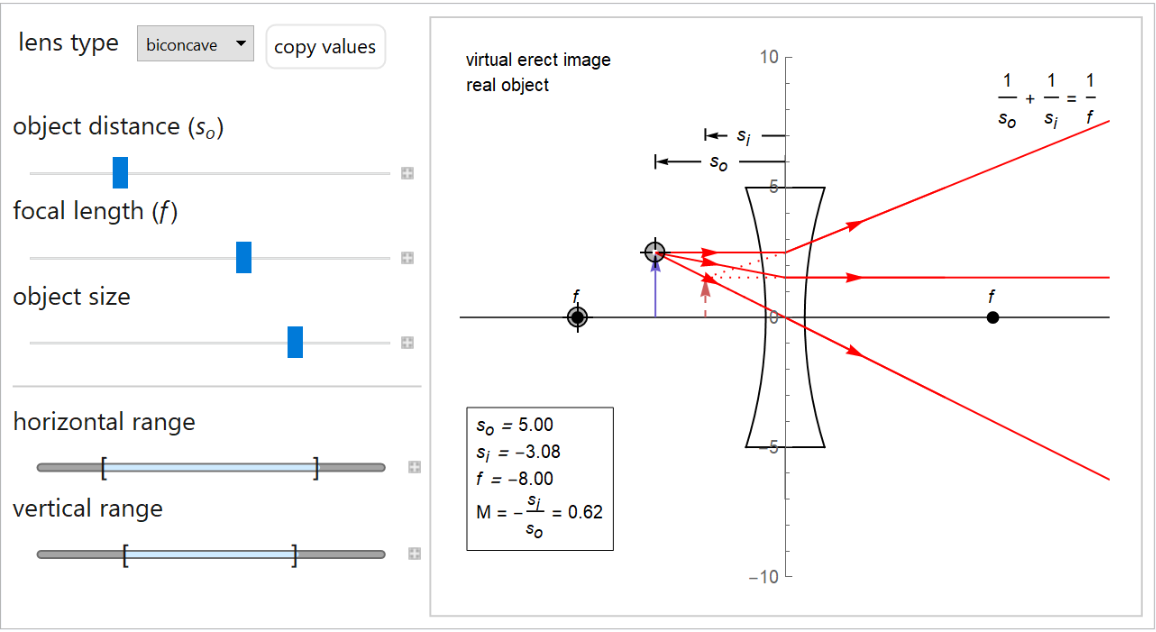### Neat Examples (1)

Customize the style:

 In:=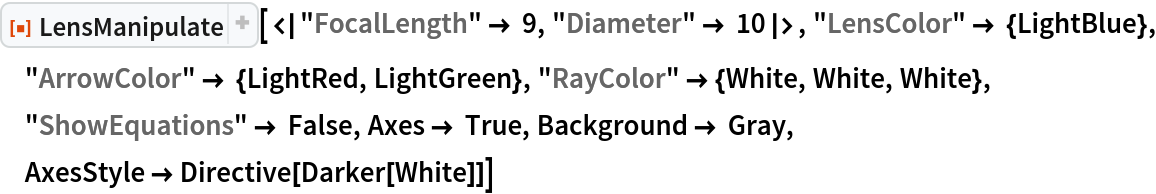Out=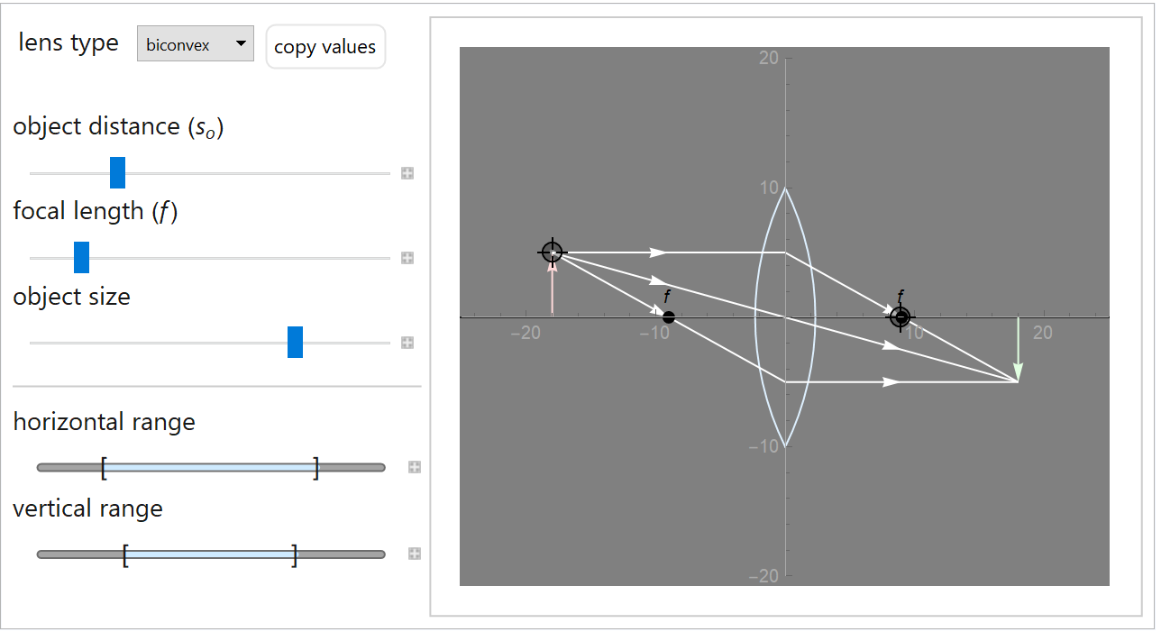## Publisher

WolframSpecialProjects

## Version History

• 1.1.0 – 12 September 2022
• 1.0.1 – 02 June 2021
• 1.0.0 – 17 March 2021